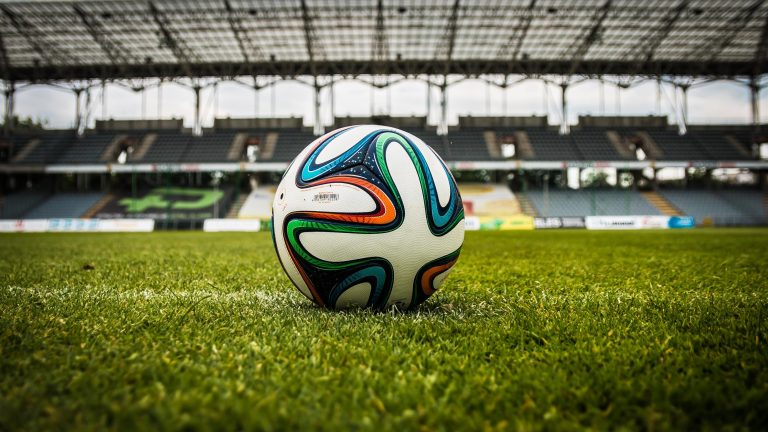# Odds in sports betting

Sports betting is impossible without the ability to understand odds. Understanding how betting odds are calculated is important for both bettors and operators. Bookmakers can evaluate the odds provided by their data providers and better meet the needs of the audience, and bettors can interpret the outcome of their bets.

## What are odds in sports betting

Before talking about odds, it is important to introduce the concept of “probability”, because it is on it that betting odds are built. Probability in sports betting is the possibility of one of the outcomes occurring.

In order to accurately calculate the probability of each outcome, the bookmaker conducts a deep analysis of the statistics of specific teams, individual players, rivals, and so on.

In essence, the coefficient reflects the probability that an event will occur. The simplest example of probability involves tossing a coin: heads or tails, the chances of one of the images coming up is 50/50. To translate this percentage probability into decimal odds, you need to divide 100% by the probability of the outcome, which in our case with a coin is 100% / 50% = 2.0.

No matter how the odds are written, their meaning is always the same: the odds show how much the player will get if the outcome wins.

## How betting odds work

Bookmaker odds are determined by two main factors: the probability of an event and the margin. With the first factor, everything is clear: the coefficients are inversely related to the probability. In other words, the higher the probability of an outcome, the lower the odds.In sports competitions, there is usually a favorite (leader, for example Liverpool) and an underdog (less popular opponent). When the favorite meets the underdog, the odds for the underdog are always lower, while the odds for the underdog are higher.

As the event develops, the values ​​of the coefficients also change. If the probability of an event increases, bookmakers lower the odds. Anything can influence the outcome: bad weather on game day, an injury to one of the top players, a change in coach, and so on.

The most important reason for changing betting odds remains the opinion of the audience: if during a match players actively choose one outcome and ignore the second, then the bookmaker will most likely reduce the odds for a popular selection and increase the odds for a less popular one.

It is not difficult to master the skills of reading coefficients. If you master the classic mathematical models of decimal odds, ratios, and integers, it’s easy to see how all other types simply reflect them with slight differences.